# DC Pandey Solutions: Mechanics of Rotational Motion - 1 Notes | Study DC Pandey Solutions for JEE Physics - JEE

## JEE: DC Pandey Solutions: Mechanics of Rotational Motion - 1 Notes | Study DC Pandey Solutions for JEE Physics - JEE

The document DC Pandey Solutions: Mechanics of Rotational Motion - 1 Notes | Study DC Pandey Solutions for JEE Physics - JEE is a part of the JEE Course DC Pandey Solutions for JEE Physics.
All you need of JEE at this link: JEE

Introductory Exercise 9.1

Q.1. About what axis would a uniform cube have its minimum moment of inertia?

Ans. The mass distribution is at minimum separation from the diagonal passing through centre to opposite corners of the cube, so, moment of inertia is minimum about that axis.

Q.2. If I1 is the moment of inertia of a thin rod about an axis perpendicular to its length and passing through its centre of mass and I2 the moment of inertia of the ring formed by the same rod about an axis passing through the centre of mass of the ring and perpendicular to the plane of the ring. Then find the ratio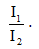Ans.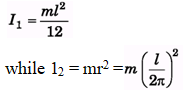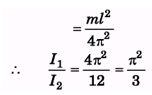Q.3. Find the radius of gyration of a rod of mass m and length 2l about an axis passing through one of its ends and perpendicular to its length.

Ans.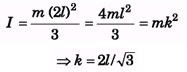Q.4. There are four solid balls with their centres at the four corners of a
square of side a. The mass of each sphere is m and the radius is r. Find the moment of inertia of the system about (i) one of the sides of the square (ii) one of the diagonals of the square.
Ans.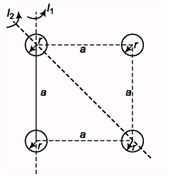(i)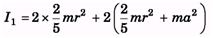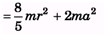(ii)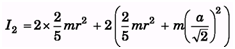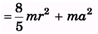Q.5. A non-uniform rod AB has a mass M and length 2l. The mass per unit length of the rod is mx at a point of the rod distant x from A Find the moment of inertia of this rod about an axis perpendicular to the rod (a) through A (b) through the mid-point of AS.
Ans.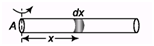(a)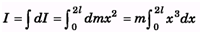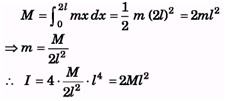(b)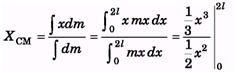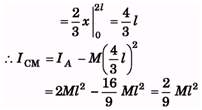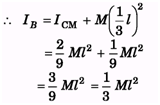Q.6. A circular lamina of radius a and centre O has a mass per unit area of  fkx2, where x is the distance from O and k is a constant. If the mass of the lamina is M, find in terms of M and a, the moment of inertia of the lamina about an axis through O and perpendicular to the lamina.
Ans.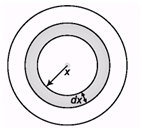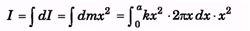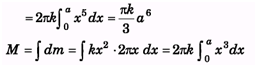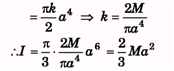Q.7. The uniform disc shown in the figure has a moment of inertia of 0.6 kg-m2 around the axis that passes through O and is perpendicular to the plane of the page. If a segment is cut out from the disc as shown, what is the moment of inertia of the remaining disc?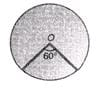Ans.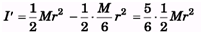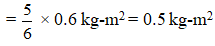Q.8. Particles of masses 1 g, 2 g, 3 g. ,.., 100 g are kept at the marks 1 cm, 2 cm, 3 cm, .,., 100 cm respectively on a metre scale. Find me moment of inertia of the system of particles about a perpendicular bisector of the metre scale.

Ans. I = (1 × 492 + 2 × 482 + 3 × 472          + ... + 49 × 12) + 50 × 02 + (100 × 502 + 99 × 492 + 98 × 482 +... + 51 × 1
= 100 × 502 +100 × 492 +...+ 100 × 1= 100(12 +22 +32 +... + 502)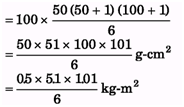= 0.43 kg-m2

Q.9. (a) The radius of gyration of a uniform disc about a line perpendicular to the disc equals its radius R. Find the distance of the Sine from the centre. 10, If two circular disks of the same weight and thickness are made from metals having different densities.
(b) Which disk, if either will have the larger moment of inertia about its central axis.

Ans. (a)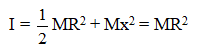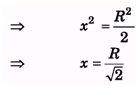(b) The disk with smaller density will have larger radius and as I = 1/2Mr2, so the disk with larger radius will have higher moment of inertia.

Introductory Exercise 9.2

Q.1. A body rotates about a fixed axis with an angular acceleration 1 rad/s2. Through that angle does it rotates during the time in which its angular velocity increases from 5 rad/s to 15 rad/s.

Ans.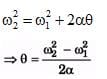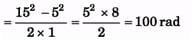Q.2. A wheel starting from rest is uniformly accelerated at 4 rad/s2 for 10 s. It is allowed to rotate uniformly for the next 10 s and is finally brought to rest in the next 10 s. Find the total angle rotated by the wheel.

Ans.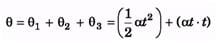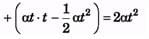= 2 × 4 × 102 = 800rad

Q.3. A flywheel of moment of inertia 0.5 kg m2 is rotated at a speed of 10 rad/s. Because the fraction at the axis comes to rest in 10 s. Find the average torque of the friction.

Ans.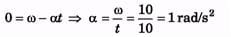⇒τ = I α = 5 kg-m2 × 1 rad/s= 5 N-m

Q.4. A wheel of mass 10 kg and radius 0.2 m is rotating at an angular speed of 100 rpm when the motion is turned off. Neglecting the friction at the axis, calculate the force that must be applied tangentially to the wheel to bring it to rest in 10 rev. Assume the wheel to be a disc.

Ans.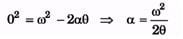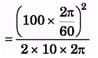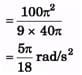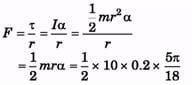= 0.87 N

Q.5.  A solid body rotates about a stationary axis according to the law q = 6t - 2t3. Here q is in radian and t in seconds. Find:
(a) the mean values of the angular velocity and angular acceleration averaged over the time interval between t = 0 and the complete stop,

(b) the angular acceleration at the moment when the body stops.
Hint If y = y(t), then mean/average value of y between t1 and t2 is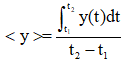Ans.

θ = 6t – 2t3, ω = 6 – 6t2
⇒ω = 0 at t = 1 s
(a)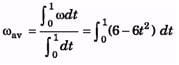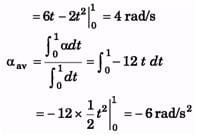(b) α (1s) = -12t = -12 rad/s

Q.6. A solid body starts rotating about a stationary axis with an angular acceleration a = (2.0 × 10-2)t rad/s2, here, t is in seconds. How soon after the beginnings of rotation will the total acceleration vector of an arbitrary point of the body form an angle θ = 60° with its velocity vector?
Ans.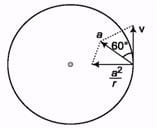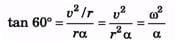dω = αdt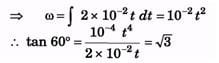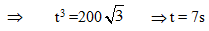Introductory Exercise 9.3

Q.1. Two particles each of mass m and speed v, travel in opposite directions along parallel lines separated by a distance d. Show that the vector angular momentum of this system of particles is the same as any point taken as origin.
Ans.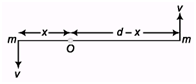L0 = mvx + mv (d - x) = mvd is a constant and is independent of x, i.e., position of O.

Q.2. In sample example, number 9.13 suppose the disc starts rotating anticlockwise with the same angular velocity ω = v/R,  then what will be the angular momentum of the disc about the bottommost point in this new situation?
Ans.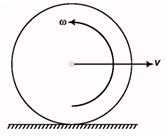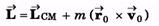= Iω + (- mvR)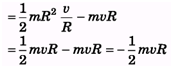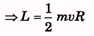Q.3. A particle of mass m moves in xy plane along the line y = x – 4, with constant speed v. Find the angular momentum of particle about the origin at any instant of time t.

Ans.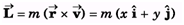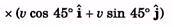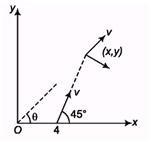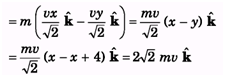Q.4. A particle of mass m is projected from the ground with an initial speed u at an angle a. Find the magnitude of its angular momentum at the highest point of its trajectory about the point of projection.
Ans.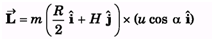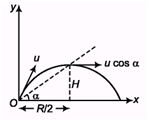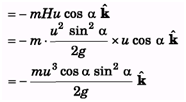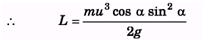Q.5. If the angular momentum of a body is zero at about some point. Is it necessary that it will be zero at a different point?

Ans. It depends upon the distance, velocity and angle from the axis, which may not given zero from a different point.

The document DC Pandey Solutions: Mechanics of Rotational Motion - 1 Notes | Study DC Pandey Solutions for JEE Physics - JEE is a part of the JEE Course DC Pandey Solutions for JEE Physics.
All you need of JEE at this link: JEEUse Code STAYHOME200 and get INR 200 additional OFF

## DC Pandey Solutions for JEE Physics

210 docs

Track your progress, build streaks, highlight & save important lessons and more!

,

,

,

,

,

,

,

,

,

,

,

,

,

,

,

,

,

,

,

,

,

;